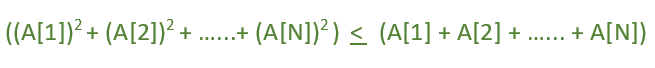# Change K elements so that (a1^2 + a2^2 + …+ aN^2 ) <= (a1 + a2 +…+ aN) becomes true

Given an array Arr of size N. The task is to tell whether it is possible to change at most K elements of this sequence to arbitrary positive integers in such a way that the below condition holds.Examples:

```Input:N = 2, Arr[] = {1, 2}, K = 2
Output: Possible
(As A can be change to 1)

Input: N = 2, Arr[] = {5, 6}, K = 1
Output: Not Possible
(As we can only change 1 element to any arbitrary number
and after changing it doesn't satisfy above condition)
```

## Recommended: Please try your approach on {IDE} first, before moving on to the solution.

Approach: When all the elements of the array becomes equal to 1 then only the given equation can be satisfied, else not.

1. Traverse the array and count the number of 1.
2. If K >= (size of array i.e N – count) then return true, Else return false.

Below is the implementation of the above approach:

## C++

 `// C++ implementation of above approach ` `#include ` `using` `namespace` `std; ` ` `  `// Function that will tell ` `// whether it is possible or Not ` `int` `Series(``int` `Arr[], ``int` `N, ``int` `K) ` `{ ` `    ``int` `count = 0; ` `    ``for` `(``int` `i = 0; i < N; i++) ` `        ``if` `(Arr[i] == 1) ` `            ``count++; ` ` `  `    ``if` `(K >= (N - count)) ` `        ``return` `1; ` `    ``else` `        ``return` `0; ` `} ` ` `  `// Driver code ` `int` `main() ` `{ ` `    ``int` `Arr[] = { 5, 1, 2 }; ` `    ``int` `N = ``sizeof``(Arr) / ``sizeof``(Arr); ` `    ``int` `K = 2; ` ` `  `    ``// Calling function. ` `    ``int` `result = Series(Arr, N, K); ` ` `  `    ``if` `(result == 1) ` `        ``cout << ``"Possible"``; ` `    ``else` `        ``cout << ``"Not Possible"``; ` `    ``return` `0; ` `} `

## Java

 `//Java  implementation of above approach  ` ` `  `import` `java.io.*; ` ` `  `class` `GFG { ` `     `  `// Function that will tell  ` `// whether it is possible or Not  ` `static` `int` `Series(``int` `Arr[], ``int` `N, ``int` `K)  ` `{  ` `    ``int` `count = ``0``;  ` `    ``for` `(``int` `i = ``0``; i < N; i++)  ` `        ``if` `(Arr[i] == ``1``)  ` `            ``count++;  ` ` `  `    ``if` `(K >= (N - count))  ` `        ``return` `1``;  ` `    ``else` `        ``return` `0``;  ` `}  ` ` `  `// Driver code  ` `    ``public` `static` `void` `main (String[] args) { ` `    ``int` `Arr[] = { ``5``, ``1``, ``2` `};  ` `    ``int` `N = Arr.length;  ` `    ``int` `K = ``2``;  ` `    ``// Calling function.  ` `    ``int` `result = Series(Arr, N, K);  ` `    ``if` `(result == ``1``)  ` `            ``System.out.println (``"Possible"``);  ` `    ``else` `            ``System.out.println( ``"Not Possible"``);  ` `         `  `    ``} ` `//This Code is Contributed by ajit     ` `} `

## Python3

 `# Python implementation of  ` `# above approach ` ` `  `# Function that will tell ` `# whether it is possible or Not ` `def` `Series(Arr, N, K): ` `    ``count ``=` `0` `    ``for` `i ``in` `range``(N): ` `        ``if` `Arr[i] ``=``=` `1``: ` `            ``count ``+``=` `1` `    ``if` `K >``=` `(N ``-` `count): ` `        ``return` `1` `    ``return` `0` ` `  `# Driver code ` `Arr ``=` `[``5``, ``1``, ``2``] ` `N ``=` `len``(Arr) ` `K ``=` `2` ` `  `result ``=` `Series(Arr, N, K) ` `if` `result ``=``=` `1``: ` `    ``print``(``"Possible"``) ` `else``: ` `    ``print``(``"Not Possible"``) ` ` `  `# This code is contributed ` `# by Shrikant13 `

## C#

 `//C# implementation of above approach ` ` `  `using` `System; ` ` `  `public` `class` `GFG{ ` `     `  `         `  `// Function that will tell  ` `// whether it is possible or Not  ` `static` `int` `Series(``int` `[]Arr, ``int` `N, ``int` `K)  ` `{  ` `    ``int` `count = 0;  ` `    ``for` `(``int` `i = 0; i < N; i++)  ` `        ``if` `(Arr[i] == 1)  ` `            ``count++;  ` ` `  `    ``if` `(K >= (N - count))  ` `        ``return` `1;  ` `    ``else` `        ``return` `0;  ` `}  ` ` `  `// Driver code  ` `     `  `    ``static` `public` `void` `Main (){ ` `    ``int` `[]Arr = { 5, 1, 2 };  ` `    ``int` `N = Arr.Length;  ` `    ``int` `K = 2;  ` `    ``// Calling function.  ` `    ``int` `result = Series(Arr, N, K);  ` `    ``if` `(result == 1)  ` `            ``Console.WriteLine (``"Possible"``);  ` `    ``else` `            ``Console.WriteLine( ``"Not Possible"``);  ` `         `  `    ``}  ` `//This Code is Contributed by akt_mit ` `}  `

## PHP

 `= (``\$N` `- ``\$count``))  ` `        ``return` `1;  ` `    ``else` `        ``return` `0;  ` `}  ` ` `  `// Driver code  ` `\$Arr` `= ``array``( 5, 1, 2 );  ` `\$N` `= sizeof(``\$Arr``);  ` `\$K` `= 2;  ` ` `  `// Calling function.  ` `\$result` `= Series(``\$Arr``, ``\$N``, ``\$K``);  ` ` `  `if` `(``\$result` `== 1)  ` `    ``echo` `"Possible"``;  ` `else` `    ``echo` `"Not Possible"``;  ` ` `  `// This code is contributed ` `// by Sach_Code ` `?> `

Output:

```Possible
```

Attention reader! Don’t stop learning now. Get hold of all the important DSA concepts with the DSA Self Paced Course at a student-friendly price and become industry ready.

My Personal Notes arrow_drop_upCheck out this Author's contributed articles.

If you like GeeksforGeeks and would like to contribute, you can also write an article using contribute.geeksforgeeks.org or mail your article to contribute@geeksforgeeks.org. See your article appearing on the GeeksforGeeks main page and help other Geeks.

Please Improve this article if you find anything incorrect by clicking on the "Improve Article" button below.